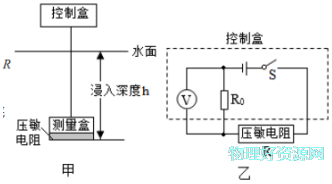# 某科技小组自制了一个测量池水深度的装置，如图甲，它主要是由控制盒(1)当测量盒浸入深度为1m时，电压表的示数为1.5V，求此时R底部受到水的压强｡

(2)当测量盒浸入深度为2m时，电压表的示数为2.0V，求电源电压和R0的阻值｡

(3)将测量盒放入水平池底（R与池底未紧密结合，导线松弛），静止时电压表示数为3V，则池水深度为多少米？

1条回答
• 解析：

(1)p=ρghR底部受到水的压强

p1=ρgh1=1×103kg/m3×10N/kg×1m=1×104Pa

(2)由图知压敏电阻RR0串联，电压表测量R0两端的电压，当h11m时，水对R底部的压力为

F1=p1S=1×104Pa×8×10-4m2=8N

查表得R1=15Ω，则有

U=I1R1+U0=R1+U0……①

h22m时，水对R底部的压力为

F2=p2S=ρgh2S=1×103kg/m3×10N/kg×2m×8×10-4m2=16N

查表得R2=10Ω，则有

U=I2R2+U0’=R2+U0……②

联立①②式代入数据解得电源电压U=6VR0=5Ω

(3)电压表示数为U3=3V时，有

U=I3R3+U3=R3+U3

代入数据解得R3=5Ω，查表得测量盒底部受到的总压力F3=48N

测量盒沉底时受到重力､支持力和浮力，其中G=2.0N

F=ρgV=1×103kg/m3×10N/kg×8×10-4m2×0.05m=0.4N

则池底对测量盒底部的支持力

F=G- F=2.0N-0.4N=1.6N

所以，水对测量盒底部的压力为

F=F3-F=48N-1.6N=46.4N

F=pS=1.0×103kg/m3×10 N/kg×h3×8×10-4m2=46.4N

解得h3=5.8m

(1)当测量盒浸入深度为1m时，电压表的示数为1.5V，求此时R底部受到水的压强为1×104Pa

(2)电源电压为6VR0的阻值为

(3)将测量盒放入水平池底（R与池底未紧密结合，导线松弛），静止时电压表示数为3V，则池水深度为5.8m

讨论(1)看不清?